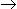# Chemical Engineering - Chemical Reaction Engineering - Discussion

43.

The dimensions of rate constant for reaction 3AB are (l/gm mole)/min. Therefore the reaction order is

 [A]. 0 [B]. 1 [C]. 2 [D]. 3

Explanation:

No answer description available for this question.

 Shekhar said: (Oct 27, 2014) Check options take c option. Rate = kc^2 Mol/L*min = k(mol/L)^2 and then solved it. K = L/MOL*MIN.

 Jomson Mathachan said: (Mar 6, 2019) General Expression for Rate Constant is (time)^(-1) * (Conctn)^(1-n). So, option C is correct.